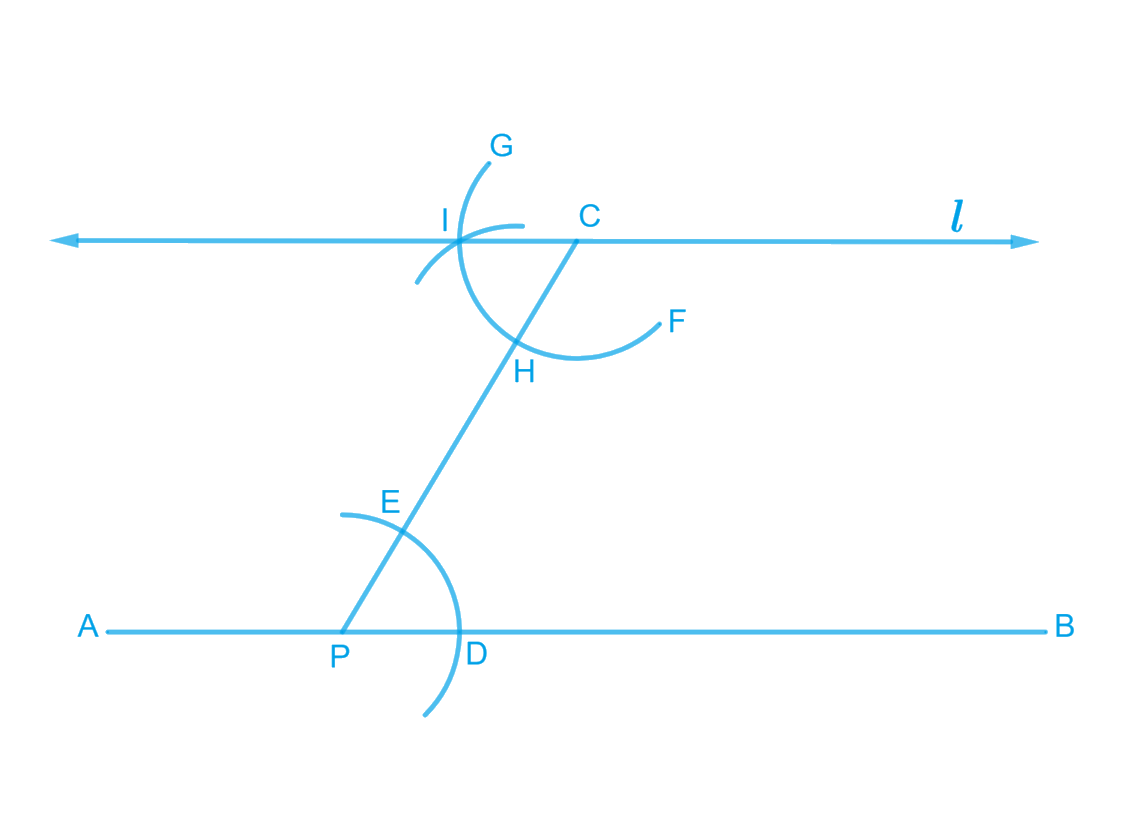# Ex.10.1 Q1 Practical Geometry Soltion - NCERT Maths Class 7

Go back to  'Ex.10.1'

## Question

Draw a line, say $$AB,$$ take a point $$C$$ outside it. Through $$C,$$draw a line parallel to $$AB$$ using ruler and compasses only.

Video Solution
Practical Geometry
Ex 10.1 | Question 1

## Text Solution

What is known?

A line $$AB$$ and a point $$C$$ outside it.

To construct:

A line through $$C$$ parallel to $$AB$$ using ruler and compasses.

Reasoning:

Draw a line $$AB$$ and take a point $$C$$ outside it. Draw line $$AB$$ by using ruler and compasses, follow the steps given below.

Steps:Steps of construction

1. Draw a line,  $$AB,$$ take a point $$C$$ outside this line. Take any point $$P$$ on $$AB$$. Join $$C$$ to $$P$$
2. Taking $$P$$ as centre and a convenient radius draw an arc intersecting line $$AB$$ at $$D$$ and $$PC$$ at $$E.$$
3. Taking $$C$$ as the centre and the same radius in previous step, draw an arc $$FG$$ intersecting $$PC$$ at $$H.$$
4. Adjust the compasses up to the length of $$DE$$. Without changing the opening of compasses and taking $$H$$ as the centre, draw an arc to intersect arc $$HG$$ at point $$I.$$
5. Join the point $$C$$ and $$I$$ to draw a line $$L.$$

This is the required line $$L$$ which is parallel to $$AB.$$

Learn from the best math teachers and top your exams

• Live one on one classroom and doubt clearing
• Practice worksheets in and after class for conceptual clarity
• Personalized curriculum to keep up with school